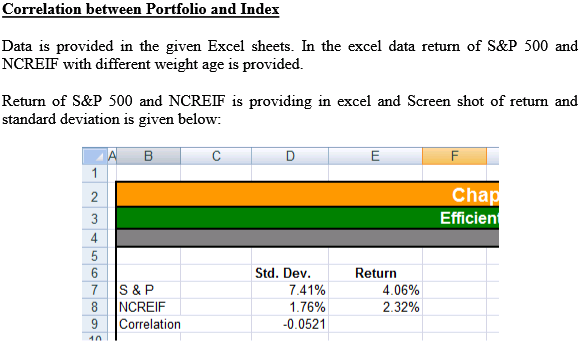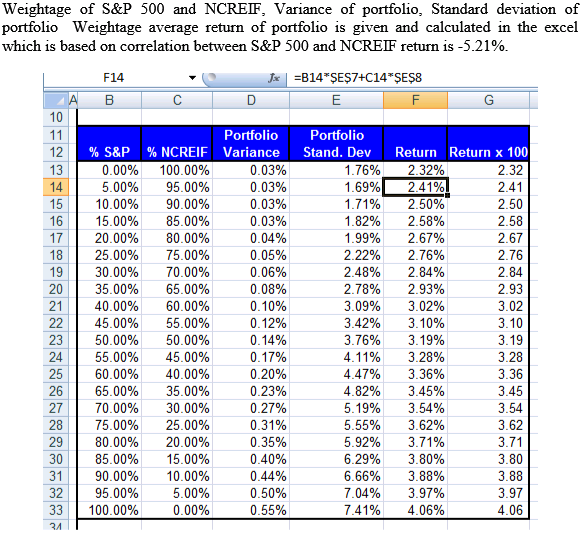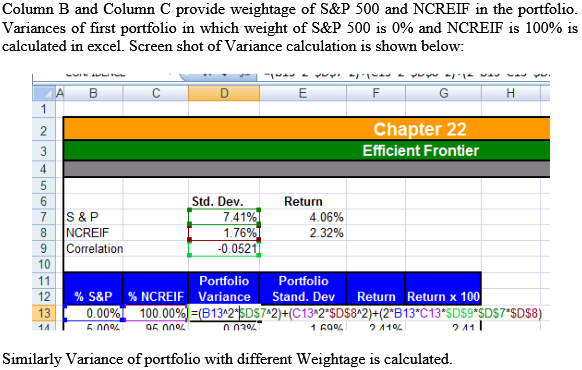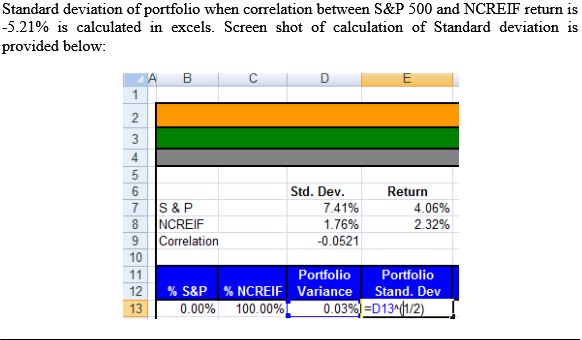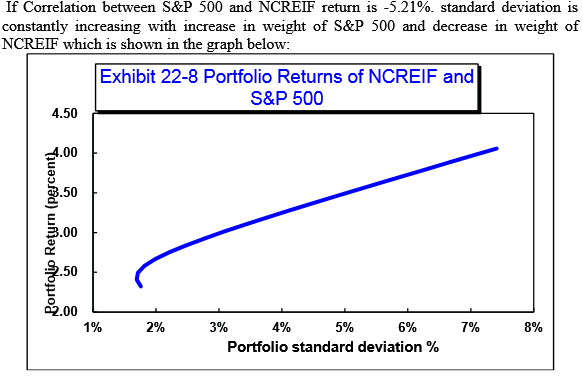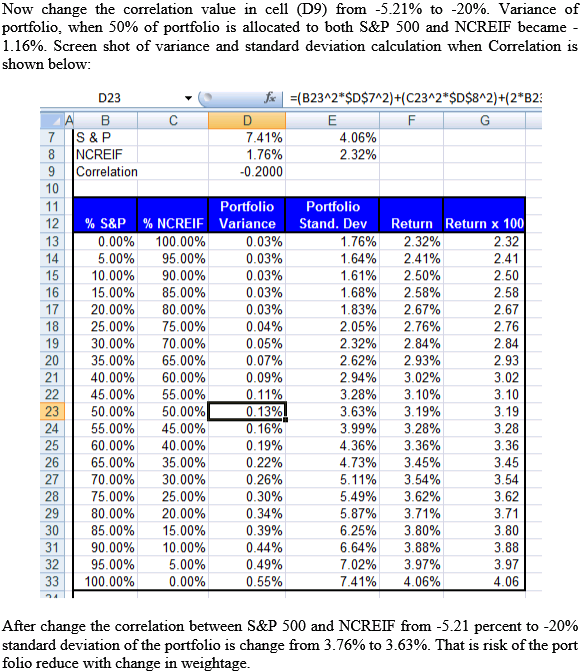# Quiz 22: Real Estate Investment Performance and Portfolio Considerations

Holding period return (HPR) is the return to the holder for the particular period of the investment of the fund. The following is the formula to calculate the holding period return: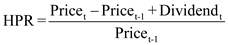Here, The holding period return is HPR. The time period is t. The price at time t is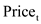. The price at time t-1 is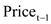The dividend at time t is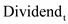. a. Quarterly holding period return (HPR) for each investment The following are the data inputs in spreadsheet: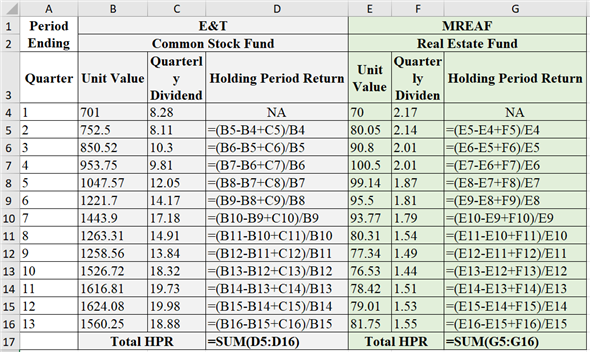The following are the obtained results in spreadsheet, the holding period return for the given values is provided below: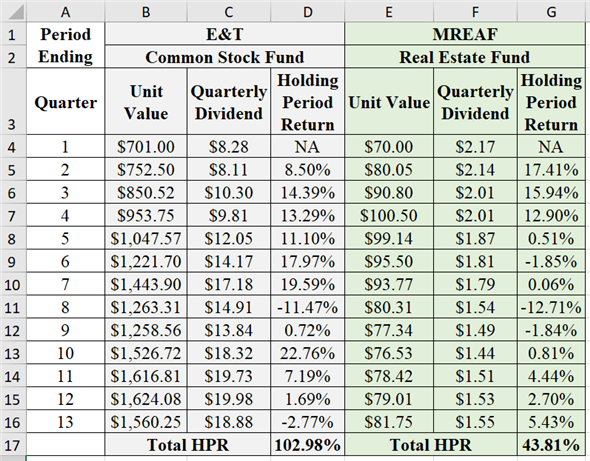b. Arithmetic mean Arithmetic mean is the sum of total values divided by the total number of values. Calculate the arithmetic mean for common stock fund as follows:Therefore, the arithmetic mean of commons stock fund is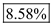. Calculate the arithmetic mean for real estate fund as follows:Therefore, the arithmetic mean of real estate fund is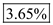. Standard deviation Standard deviation is a measure of dispersion of possible outcomes around the expected value of a random variable. Standard deviation is calculated as follows:Where, The variance is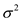. The each value in the set is X. The arithmetic mean is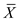The number of return is N. Standard deviation of common stock funds' HPR: The following are the data inputs in spreadsheet: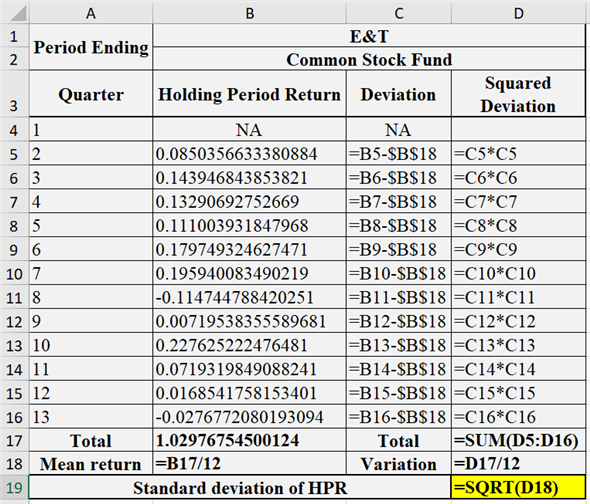The following are the obtained results in spreadsheet: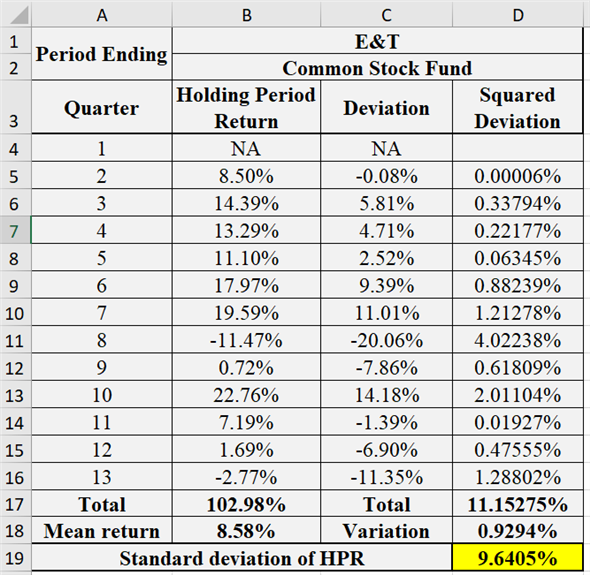Therefore, the standard deviation of common stock funds' HPR is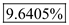. Standard deviation of real estate funds' HPR: The following are the data inputs in spreadsheet: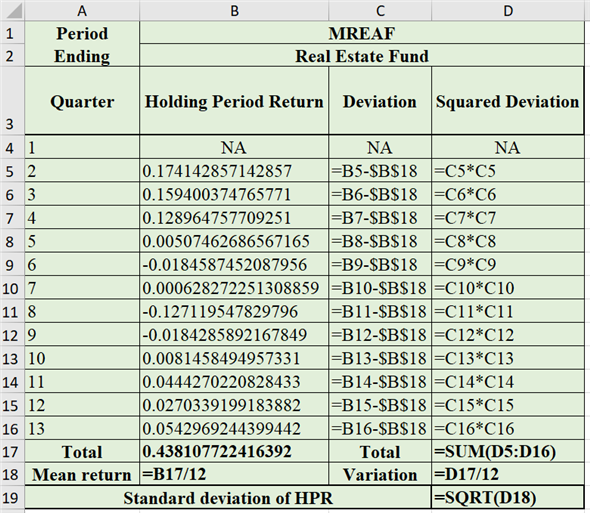The following are the obtained results in spreadsheet: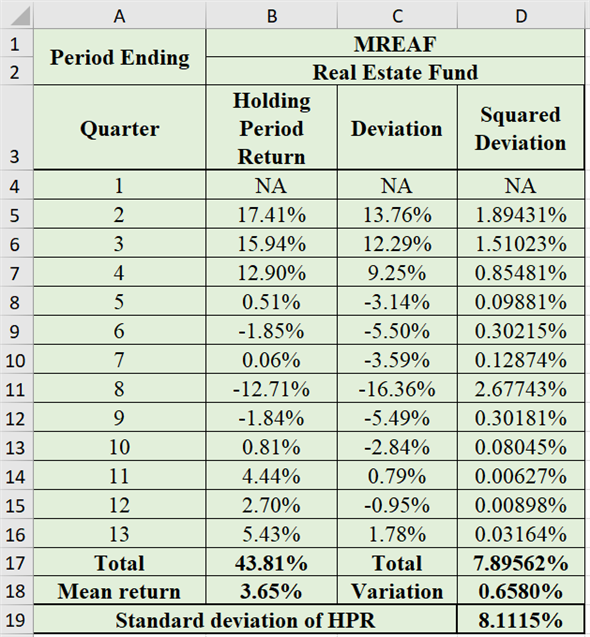Therefore, the standard deviation of real estate funds' HPR is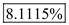. Geometric mean Geometric mean is the measure of compound rate of growth over the period of time. It assumes that all cash flows are reinvested in the asset and those reinvested fund earn the following rates on return. Calculate the geometric mean of common stock as follows: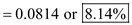Therefore, the geometric mean of common stock is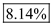. Calculate the geometric mean of common stock as follows: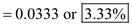Therefore, the geometric mean of real estate fund is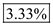. Risk per unit: Coefficient of variation Calculate the coefficient of variation of common stock as follows:Therefore, the coefficient of variation of common stock fund is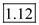. Calculate the coefficient of variation of real estate fund as follows:Therefore, the coefficient of variation of real estate fund is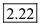. The real estate fund has more coefficient of variation. Hence, real estate fund contains more risk per unit as compared to the common stock fund. c. Correlation The covariance between the securities of a portfolio measures the relationship between the securities, it may be negative, positive or zero. The positive covariance between the securities increase the risk of the portfolio therefore the negative covariance is always preferable by the investors. The following are the data inputs in spreadsheet: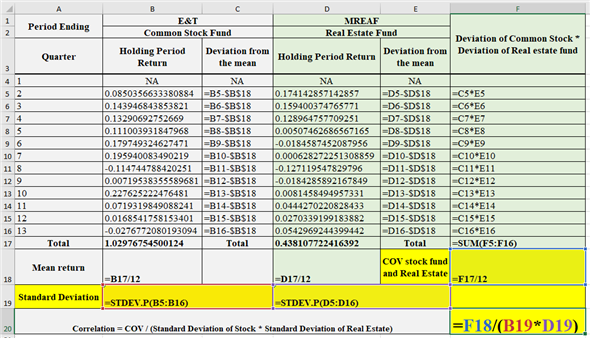The following are the obtained results in spreadsheet: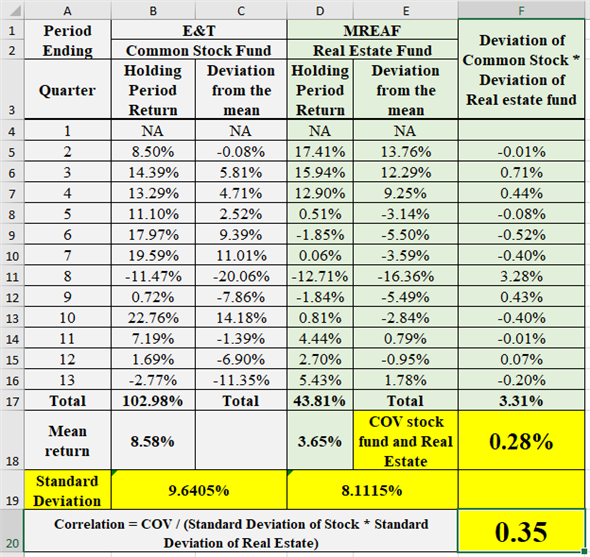Therefore, the correlation between the common stock fund and real estate fund is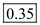. d. 50% of each fund provided any diversification or not The following are the data inputs in spreadsheet: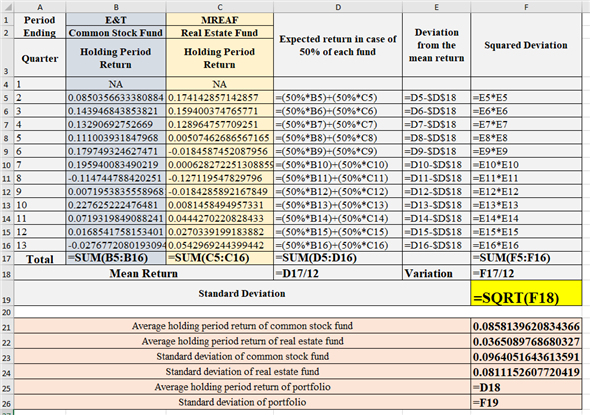The following are the obtained results in spreadsheet: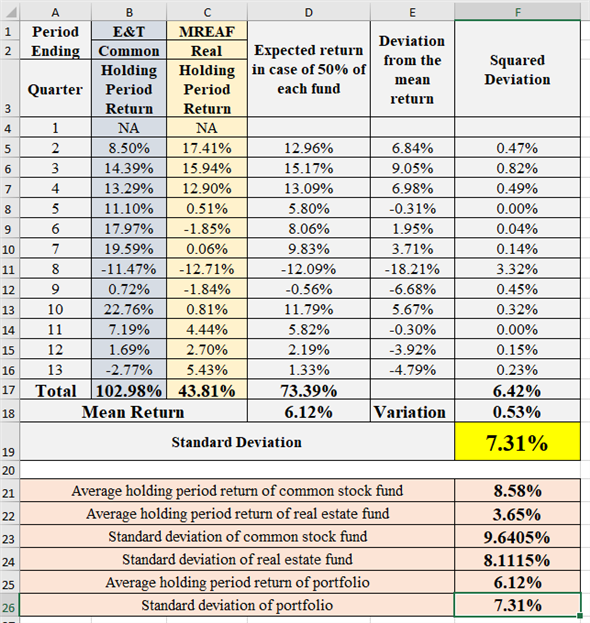Therefore, it is clear from the above obtained results that the portfolio of containing 50% of common stock fund and 50% of real estate fund provided diversification and reduced the overall standard deviation (risk) of the investment. e. Optional f. If the manager of ET T is considering for the investment in MREAF then this analysis is very helpful in that as this analysis will show the risk per unit in terms of coefficient of covariation and it will show the risk as standard deviation. If the investment is done in the proportion/weightage then it will show the portfolio's expected return and standard deviation which will be very helpful in making decision and deciding the optimal asset allocation through this analysis only.